## A child on a sled has a combined mass of 97 kg. At the top of a 3.1 meter hill, the sled has a velocity of 8 m/s. Assuming there is no frict

Question

A child on a sled has a combined mass of 97 kg. At the top of a 3.1 meter hill, the sled has a velocity of 8 m/s. Assuming there is no friction, what would the velocity of the child and sled be when they reach the top of a different 1.2 meter hill?

in progress 0
6 months 2021-08-01T16:16:32+00:00 1 Answers 5 views 0

Explanation:

This is an energy conservation problem in that the total energy available to a system s constant trhoughout the whole problem. That is, in equation form:

TE = PE + KE. This is a bit of a problem due to sig figs here, but I will keep with the rules for them all the way up til the end of the problem (I’ll tell you when I veer away from the rule and why I did when I get there).

We first need to find the total energy available to the system and then use that value throughout the rest of the problem.

TE = PE + KE where

PE = mgh (mass times gravity times height of the object) and

KE =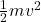(one-half times the mass of the object times the square of the velocity of the object). We solve for PE first, rounding to the correct number of sig figs:

PE = 97(9.8)(3.1) and

PE = 2900 J (2 sig figs here since all the numbers given have 2 sig figs in them). Now for KE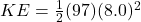which, to 2 sig figs (notice I added a .0 to the 8), is

KE = 2200. This means that the total energy available to the sled throughout the whole trip is

TE = 2900 + 2200 so

TE = 5100 J

Now for the second part of the problem, the sled is at a different height so the PE is different, and we are asked to find the velocity at the top of this second hill. The total energy equation then is

5100 = PE + KE. Solving for PE first:

PE = (97)(9.8)(1.2) and, to 2 sig figs:

PE = 82. Now for the KE: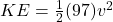. Plugging all of that into the total energy equation gives us: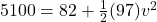and isolating the v: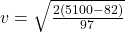and this is where I veer away from the sig figs. Doing the subtracting first, as we should, means that we would round to the hundreds place. Since the least significant place in the number 82 is the tens place and the least significant place in the 5100 is in the hundreds place, we round to the hundreds place. But I didn’t keep to this rule because I’m not sure how adamant your physics teacher is about sig figs. I’ll give you the answer that I would expect from my students (the right one) after I give you this one. I just simply subtracted 5100 – 82 and used the answer the calculator gave me which was 5018: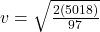gives us that the velocity at the top of the 1.2 meter hill is

10.2 m/s.

Doing this the right way, using the rules for sig figs properly: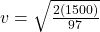gives us, to 2 sig figs,

v = 1.0 × 10¹ m/s